Courses

# Graphs Theory MCQ - 2

## 20 Questions MCQ Test RRB JE for Computer Science Engineering | Graphs Theory MCQ - 2

Description
This mock test of Graphs Theory MCQ - 2 for Computer Science Engineering (CSE) helps you for every Computer Science Engineering (CSE) entrance exam. This contains 20 Multiple Choice Questions for Computer Science Engineering (CSE) Graphs Theory MCQ - 2 (mcq) to study with solutions a complete question bank. The solved questions answers in this Graphs Theory MCQ - 2 quiz give you a good mix of easy questions and tough questions. Computer Science Engineering (CSE) students definitely take this Graphs Theory MCQ - 2 exercise for a better result in the exam. You can find other Graphs Theory MCQ - 2 extra questions, long questions & short questions for Computer Science Engineering (CSE) on EduRev as well by searching above.
QUESTION: 1

### If G is a forest with n vertices and k connected components, how many edges does G have?

Solution:

Each component will have n/k vertices (pigeonhole principle). Hence, for each component there will be (n/k)-1 edges. Since there are k components, total number of edges= k*((n/k)-1) = n-k.

QUESTION: 2

### Let d denote the minimum degree of a vertex in a graph. For all planar graphs on n vertices with d ≥ 3, which one of the following is TRUE?

Solution:

Euler's formula for planar graphs:

v − e + f = 2.

v => Number of vertices
e => Number of edges
f => Number of faces

Since degree of every vertex is at least 3, below is true from handshaking lemma (Sum of degrees is twice the number of edges)

3v >= 2e
3v/2 >= e

Putting these values in Euler's formula.
v - 3v/2 + f >= 2

f >= v/2 + 2

QUESTION: 3

### The 2n vertices of a graph G corresponds to all subsets of a set of size n, for n >= 6 . Two vertices of G are adjacent if and only if the corresponding sets intersect in exactly two elements. The number of vertices of degree zero in G is:

Solution:

There are n nodes which are single and 1 node which belong to empty set. And since they are not having 2 or more elements so they won’t be connected to anyone hence total number of nodes with degree 0 are n+1 hence answer should be none. Thanks to roger for the explanation.

QUESTION: 4

The 2n vertices of a graph G corresponds to all subsets of a set of size n, for n >= 6. Two vertices of G are adjacent if and only if the corresponding sets intersect in exactly two elements. The number of connected components in G is:

Solution:

n+1 nodes of the graph not connected to anyone as explained in question 70 while others are connected so total number of connected components are n+2 (n+1 connected components by each of the n+1 vertices plus 1 connected component by remaining vertices)

QUESTION: 5

Let G be a simple connected planar graph with 13 vertices and 19 edges. Then, the number of faces in the planar embedding of the graph is

Solution:

An undirected graph is called a planar graph if it can be drawn on a paper without having two edges cross and such a drawing is called Planar Embedding. We say that a graph can be embedded in the plane, if it planar. A planar graph divides the plane into regions (bounded by the edges), called faces. Graph K4 is palanar graph, because it has a planar embedding as shown in figure below.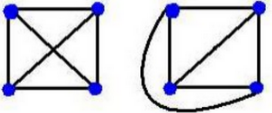Euler's Formula : For any polyhedron that doesn't intersect itself (Connected Planar Graph),the • Number of Faces(F) • plus the Number of Vertices (corner points) (V) • minus the Number of Edges(E) , always equals 2. This can be written: F + V − E = 2. Solution : Here as given, F=?,V=13 and E=19 -> F+13-19=2 -> F=8 So Answer is (B).

QUESTION: 6

Let G be a simple graph with 20 vertices and 100 edges. The size of the minimum vertex cover of G is 8. Then, the size of the maximum indepen­dent set of G is

Solution:

Background Explanation: Vertex cover is a set S of vertices of a graph such that each edge of the graph is incident to at least one vertex of S. Independent set of a graph is a set of vertices such that none of the vertices in this set have an edge connecting them i.e. no two are adjacent. A single vertex is an independent set, but we are interested in maximum independent set, that is largest set which is independent set. Relation between Independent Set and Vertex Cover : An interesting fact is, the number of vertices of a graph is equal to its minimum vertex cover number plus the size of a maximum independent set. How? removing all vertices of minimum vertex cover leads to maximum independent set. So if S is the size of minimum vertex cover of G(V,E) then the size of maximum independent set of G is |V| - S. Solution: size of minimum vertex cover = 8 size of maximum independent set = 20 - 8 =12 Therefore, correct answer is (A). References : vertex cover maximum independent set.

QUESTION: 7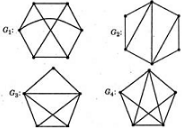Which one of the following graphs is NOT planar?

Solution:

A graph is planar if it can be redrawn in a plane without any crossing edges. G1 is a typical example of nonplanar graphs.

QUESTION: 8

Let s and t be two vertices in a undirected graph G + (V, E) having distinct positive edge weights. Let [X, Y] be a partition of V such that s ∈ X and t ∈ Y. Consider the edge e having the minimum weight amongst all those edges that have one vertex in X and one vertex in Y. Let the weight of an edge e denote the congestion on that edge. The congestion on a path is defined to be the maximum of the congestions on the edges of the path. We wish to find the path from s to t having minimum congestion. Which one of the following paths is always such a path of minimum congestion?

Solution:

Suppose shortest path from A->B is 6, but in MST, we have A->C->B (A->C = 4, C->B = 3), then along the path in MST, we have minimum congestion, i.e 4

QUESTION: 9

The minimum number of colours required to colour the following graph, such that no two adjacent vertices are assigned the same colour, is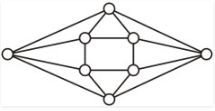Solution: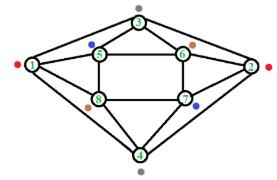Two vertices are said to be adjacent if they are directly connected, i.e., there is a direct edge between them. So, here, we can assign same color to 1 & 2 (red), 3 & 4 (grey), 5 & 7 (blue) and 6 & 8 (brown). Therefore, we need a total of 4 distinct colors. Thus, C is the correct choice.

Please comment below if you find anything wrong in the above post.

QUESTION: 10

Let G be an arbitrary graph with n nodes and k components. If a vertex is removed from G, the number of components in the resultant graph must necessarily lie between

Solution:

Minimum: The removed vertex itself is a separate connected component. So removal of a vertex creates k-1 components. Maximum: It may be possible that the removed vertex disconnects all components. For example the removed vertex is center of a star. So removal creates n-1 components.

QUESTION: 11

How many perfect matchings are there in a complete graph of 6 vertices ?

Solution:

A perfect matching, every vertex of the graph is incident to exactly one edge of the matching. A perfect matching is therefore a matching of a graph containing n/2 edges, the largest possible, meaning perfect matchings are only possible on graphs with an even number of vertices.

QUESTION: 12

A graph G = (V, E) satisfies |E| ≤ 3 |V| - 6. The min-degree of G is defined as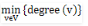Therefore, min-degree of G cannot be

Solution:

Let the min-degree of G be x, then G has at least |v| *x/2 edges. |v|*x/2 <= 3* |v| -6 for x=6, we get 0 <= -6, Therefore, min degree of G cannot be 6. Hence answer is (D).

QUESTION: 13

The minimum number of colours required to colour the vertices of a cycle with η nodes in such a way that no two adjacent nodes have the same colour is

Solution:

We need 3 colors to color a odd cycle and 2 colors to color an even cycle.

QUESTION: 14

Maximum number of edges in a n - node undirected graph without self loops is

Solution:

Background required - Basic Combinatorics Since the given graph is undirected, that means the order of edges doesn't matter. Since we have to insert an edge between all possible pair of vertices, therefore problem reduces to finding the count of the number of subsets of size 2 chosen from the set of vertices. Since the set of vertices has size n, the number of such subsets is given by the binomial coefficient C(n,2) (also known as "n choose 2"). Using the formula for binomial coefficients, C(n,2) = n(n-1)/2.

QUESTION: 15

Let G be a connected planar graph with 10 vertices. If the number of edges on each face is three, then the number of edges in G is _______________.

Solution:

Euler's formula states that if a finite, connected, planar graph is drawn in the plane without any edge intersections, then

v − e + f = 2.
v -> Number of vertices
e -> Number of edges
f -> Number of faces

As per the question v = 10 And number of edges on each face is three Therefore, 2e = 3f [Note that every edge is shared by 2 faces]

Putting above values in v − e + f = 2
10 - e + 2e/3 = 2
e = 3*10 - 6 = 24

QUESTION: 16

A graph is self-complementary if it is isomorphic to its complement. For all self-complementary graphs on n vertices, n is

Solution:

An n-vertex self-complementary graph has exactly half number of edges of the complete graph, i.e., n(n − 1)/4 edges, and (if there is more than one vertex) it must have diameter either 2 or 3. Since n(n −1) must be divisible by 4, n must be congruent to 0 or 1 mod 4; for instance, a 6-vertex graph cannot be self-complementary.

QUESTION: 17

In a connected graph, a bridge is an edge whose removal disconnects a graph. Which one of the following statements is True?

Solution:

A bridge in a graph cannot be a part of cycle as removing it will not create a disconnected graph if there is a cycle.

QUESTION: 18

What is the number of vertices in an undirected connected graph with 27 edges, 6 vertices of degree 2, 3 vertices of degree 4 and remaining of degree 3?

Solution:

The idea is to use Handshaking Lemma :- In any graph, the sum of all the vertex-degree is equal to twice the number of edges.

Let x = Number of vertices of degree 3.
By Handshaking Lemma
6*2 + 3*4 + (x-9)*3 = 27*2
24 + (x-9)*3 = 54
x-9 = 10
x = 19

QUESTION: 19

The minimum number of colours that is sufficient to vertex-colour any planar graph is _______________
[This Question was originally a Fill-in-the-blanks Question]

Solution:

A planar graph is a graph on a plane where no two edges are crossing each other. The set of regions of a map can be represented more abstractly as an undirected graph that has a vertex for each region and an edge for every pair of regions that share a boundary segment. Hence the four color theorem is applied here. Here is a property of a planar graph that a planar graph does not require more than 4 colors to color its vertices such that no two vertices have same color. This is known four color theorem.

QUESTION: 20

If all the edge weights of an undirected graph are positive, then any subset of edges that connects all the vertices and has minimum total weight is a

Solution:

As here we want subset of edges that connects all the vertices and has minimum total weight i.e. Minimum Spanning Tree Option A - includes cycle, so may or may not connect all edges. Option B - has no relevance to this question. Option C - includes cycle, so may or may not connect all edges. Related: